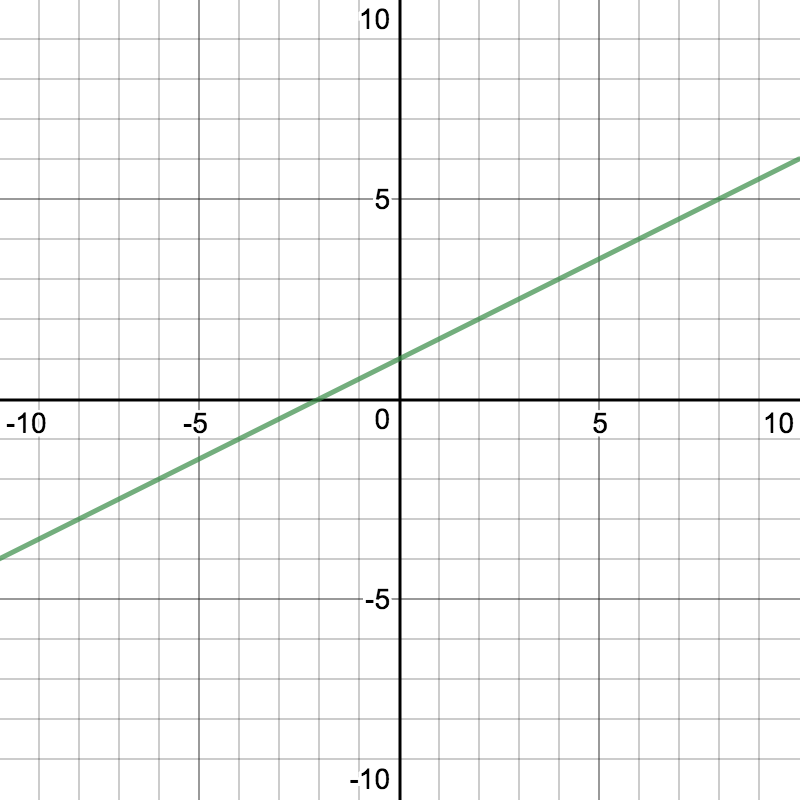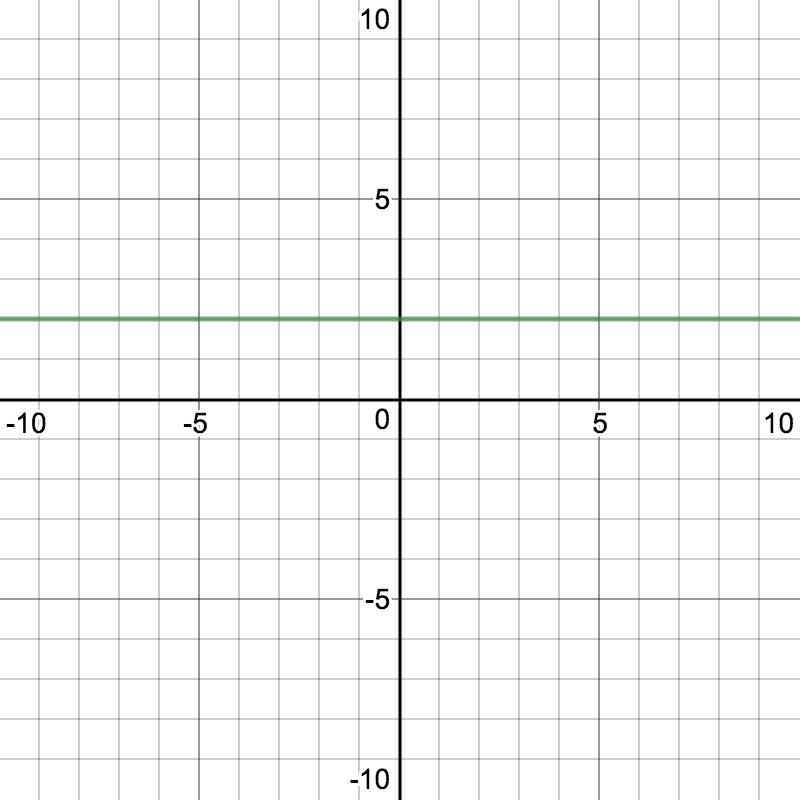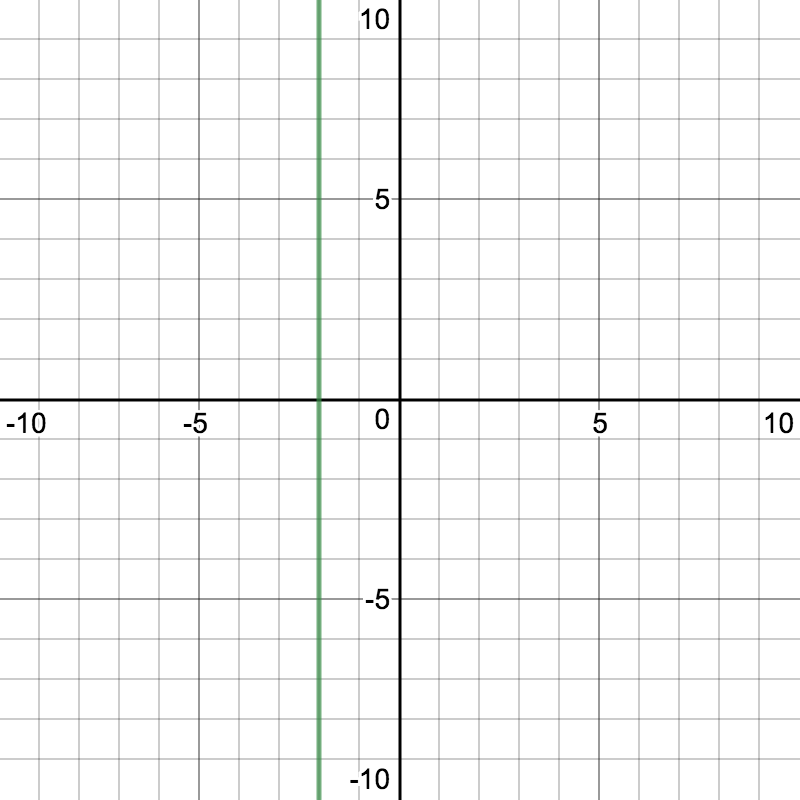# Matching Graphs to Equations

In Lesson 3 you graphed equations of lines and you wrote equations of lines in slope intercept form: $$y=mx+b$$.  Also you found that the equation of a horizontal line is of the form $$y=b$$ and that a vertical line is of the form $$x = a$$, where $$a$$ and $$b$$ are constants.

Below you will see equations on the left and graphs on the right.  Match the equation on the left to the graph of the line on the right.

 1.   $$y=-2x+1$$ A.2.   $$x=-2$$ B.3.   $$y=2$$ C.4.   $$y=\dfrac{1}{2}x+1$$ D.5.  $$y=-\dfrac{2}{3}x-1$$ E.Check solutions here.